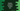# How to print Geometric progression or GP in python## Python program to print Geometric progression or GP:

In this post, we will learn how to print the Geometric progression or GP in Python. We will take the first number, common ratio for the GP and total number of elements(n) to print in the series.

### How Geometric progression works:

Let’s try to understand how Geometric progression works. Geometric progression or Geometric session or GP is a series of numbers where each number is calculated by multiplying the previous number by a constant value. This constant value is called common ratio.

For example, 5, 10, 20, 40… is a Geometric progression with common ratio 2.

If a is the starting number and r is common ratio, then a Geometric progression looks like a, ar, ar^2, ar^3….

So, for the nth number in a Geometric progression is a * r^(n - 1). To print a Geometric progression, we can take the first number, value of common ratio and total numbers to print as inputs from the user.

Let me show you the algorithm that we will use to write the program.

## Algorithm for Geometric progression:

Below algorithm we will use to print a GP:

• Take the value of the start number, common ratio and total numbers to print as inputs from the user.
• Run a loop to print the series for total numbers of time.

• Assign start number to a variable. This variable will hold the last value of the series.
• Print the last value variable.
• Update the last value variable by multiplying it with common ratio.
• Move to the next iteration.
• Once the loop ends, exit from the program.

## Python program:

Below is the complete python program to print Geometric progression:

``````def print_geometric_progression(a, r, n):
current_value = a

for i in range(n):
print(current_value, end=' ')
current_value = current_value * r

a = int(input('Enter the first element: '))
r = int(input('Enter the common ratio: '))
n = int(input('Enter total numbers to print: '))

print_geometric_progression(a, r, n)``````

Here,

• We are taking the first element as user input and storing that in the variable a. Similarly, common ratio is stored in r and total numbers is stored in n.
• printgeometricprogression method is used to print the geometric progression. It takes a, r, and n as its parameters.

• It keeps the value of a in current_value variable, which is the value to print.
• The for loop runs for n number of times.
• On each iteration, it prints current_value and changes it to current_value * r.

### Output:

If you run this program, it will print output as like below:

``````Enter the first element: 4
Enter the common ratio: 3
Enter total numbers to print: 5
4 12 36 108 324``````